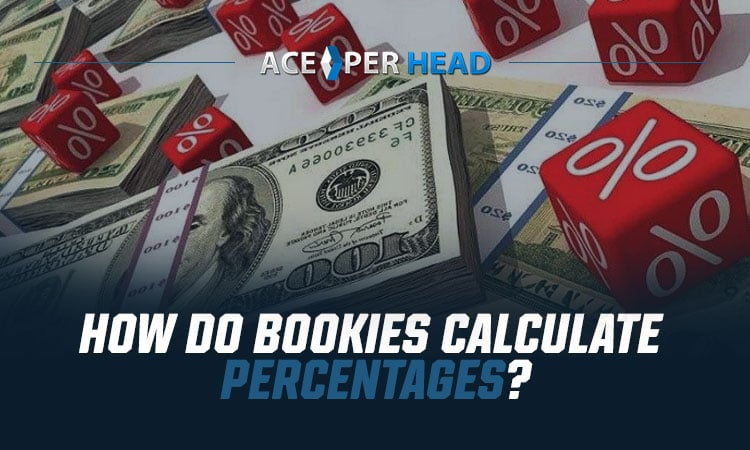When bookmakers set their markets for a race or another type of sporting event, their goal is to turn a profit, no matter which team or competitor wins.

To do so, they have to perfect the art of calculating the book percentage. This can be a tricky step that involves a few different variables and moving parts. In today’s betting guide, we’re sharing how it works and what prospective bookies need to know.

## Understanding Book Percentage

Any time you discuss the probability that a specific event will happen, you express that likelihood in terms of a percentage. For instance, if you toss a penny into the air, there’s a 50% chance that it will land on heads and a 50% chance that it will land on tails.

In that case, the book percentage is 100%. In sports betting, we call that a perfect book. However, that’s a simplified example.

It gets a little trickier when you consider all of the possibilities that could occur in a given sporting event. Trying to identify all of those correct odds and adding them up to make 100% can be daunting.

When a bookie prices up any type of event, they always factor their own profit into the odds. In the betting realm, this is called an overground.

Naturally, when you add those bookmaker percentages into the mix, the cumulative odds will be greater than 100%. In fact, in an average race, the bookmaker’s odds can be upward of 120% or more, depending on the number of participants.

If there aren’t too many runners, the odds will be lower. This is also the case when there are a few prized horses that are favored to win, and the odds aren’t as evenly spread out.

## Calculating Book Percentage

Technically speaking, the book percentage is equal to the reciprocal decimal odds, all added together. Take a horse race, for example. Bookies will take the decimal odds for each horse and divide it into 1. This gives them the reciprocal decimal odd for each horse.

For instance, if the decimal odd is 0.5, they would perform this calculation: 1/0.5. This gives the reciprocal decimal odd of 2.

Once they’ve done this for all of the odds, they’ll then add all of the numbers together. Unfortunately, they can’t just add all of the decimal odds up and then divide the grand total into 1. They have to calculate each reciprocal decimal odd individually.

### Racing Example

This can be confusing, so let’s work it into a real-life example of a 10-runner race. To find the book percentage of a race, divide 1 by each of these decimal odds. Then, add the sums together.

• Runner 1: 6 (1/6) = .166
• Runner 2: 6 (1/6) = .166
• Runner 3: 6.5 (1/6.5) = .154
• Runner 4: 7 (1/7) =.143
• Runner 5: 7.5 (1/7.5) = .133
• Runner 6: 7.5 (1/7.5) = .133
• Runner 7: 8 (1/8) = .125
• Runner 8: 8 (1/8) = .125
• Runner 9: 8.5 (1/8.5) = .118
• Runner 10: 12 (1/12) = .083

Now, add them all up. This gives us a sum of 1.346. To get the book percentage, the last step is to multiply this number by 100, which gives us 134.6%. The bookie’s profit on this race, despite who wins, should be 34.6% if the bets are properly balanced.

### Calculate Percentages With This Betting Guide

Now that you know a little more about how bookies calculate their book percentages, it’s easier to understand the sports betting world. If you want to learn more about this exciting and fast-paced field, Ace Pay Per Head has the resources you need to get started.

For tools like this betting guide and much more, sign up today! You could get up to six weeks free as you try out Ace Per Head services and learn the tools of the trade.